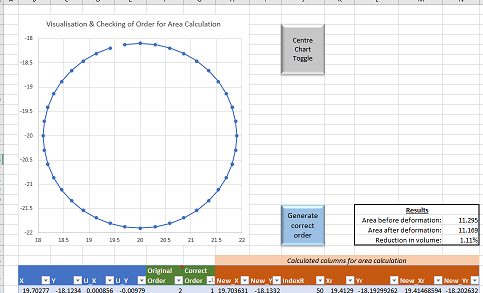# Volume loss due to tunnelling: Calculating volume loss directly from tunnel deformation in PlaxisDownload Plaxis volume loss spreadsheet – when you open this file, a yellow bar might appear below the ribbon menu asking to enable macros, click “enable content” so that the buttons on spreadsheet can work.

### What is it?

The spreadsheet calculates volume loss in a tunnel based on its deformation in Plaxis. The spreadsheet calculates volume loss (%) as 1-A/B where A = cross-sectional area of the deformed tunnel and B = cross-sectional area of the non-deformed tunnel. This method is different to the standard method of calculating volume loss from surface deformation.

### Why have I created it?

Calculating volume loss at the surface is usually done by assuming a Gaussian settlement curve, measuring the maximum surface deformation, taking the depth to tunnel axis and estimating the trough width parameter (K). This method will yield slightly different results compared to the spreadsheet. The reasons may be real, with the volume loss at the surface different to the volume loss in the tunnel due to volumetric strain in the soil. The differences can also be due to difficulties in modelling the surface deformation or assuming an incorrect K value. This spreadsheet can be used for cross-referencing your results or if need be; estimating K by back-calculation.

### What information does the spreadsheet require?

Tunnels (in Plaxis) are often modelled with plate elements of 0 thickness forming the tunnel lining. After running the model, a table that contains positional information on the nodes that represent the plate elements can be obtained from the calculated results. This is shown below:The table in Plaxis contains information on each node: that is the X and Y co-ordinates of the nodes and the distances they have deformed (X, Y & total distance). The table in the spreadsheet only requires columns 4 – 7  (X, Y, Ux & Uy) for calculation. The quickest method is to use Ctrl + A to select everything and Ctrl + C to copy from the Plaxis table. In the spreadsheet: paste the data outside of the table, select the 4 required columns and then copy and paste those columns into the table.

### How does the spreadsheet work?

To calculate the cross-sectional areas based on X and Y co-ordinates, the Shoelace formula is used which requires the nodes to be in the correct order. Unfortunately the output table in Plaxis does not have the nodes in the correct order so I created a macro that re-orders the nodes in a table in Excel using their non-deformed X and Y co-ordinates. It works by finding the two nodes that are furthest apart, taking one of those nodes and then uses a nearest neighbour algorithm to order the remaining nodes. The spreadsheet also contains a scatter chart that has markers representing each node which are connected to one another based on their order. This makes it easy to check if the macro has run properly.The shoelace formula assumes that straight lines connect each node; this very slightly underestimates the cross-sectional areas of both the deformed tunnel and the non-deformed tunnel. However, taking this level of detail into consideration would unrealistically assume a very high level of accuracy in the model. Also the effect on volume loss (%) is negligible as it is based on the ratio of both tunnels cross-sectional areas.

### Couldn’t a simpler method be used?

The spreadsheet might come across as excessive in obtaining the deformed and non-deformed cross-sectional areas of a tunnel as it requires a macro to reorder the nodes. Another formula that could be used (this is for figuring out the cross sectional area of the deformed circular tunnel) is$\frac{\pi}{t} \sum_{n=1}^{t} (R-|u|_{n})^2$. Where R is the radius, |u| is the distance deformed for each node and t is the total number of nodes.  Although this is a simpler method and doesn’t require the nodes to be reordered, it only works with the following assumptions:

1. The deformations are so small that it is safe to assume that direction of the deformations are always towards the tunnel axis even when σh ≠ σv .
2. The movement of the tunnel axis is negligible.
3. The distances between each adjacent node are equal.
4. The tunnel has circular geometry.

Point 1 is true. In most cases point 2 is true, although flotation of the tunnel might be an issue. Point 3 is false, as many nodes are duplicates, and thus some nodes would be weighted unfairly (although admittedly, the duplicates are spread out over the tunnel so this does not seem to cause too much of an issue).

The spreadsheet uses the shoelace formula instead as it is more robust, more accurate and is also applicable to non-circular geometry.

1.•2.3.